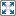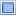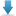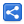# Java 8 Streams - Reduction

[Last Updated: Apr 29, 2017]

Reduction operations, in general, are terminal operations which combine stream elements and return a summary result either as:

• A single value by using `reduce()`, or it's special cases: `min()`, `max()`, `count()`, `sum()`, `average()`, `summaryStatistics()`.
• Or a collection by using `collect()` or `toArray()` methods. These methods are categorize as mutable reduction because it collects desired result into a mutable object such as a Collection.

In this tutorial we are going to go through reduce() methods. We will explore collect() methods (mutable reduction) in the next tutorial.

## Stream#reduce() methods

These methods can generally be described as fold operations. A fold operation uses a binary function (accumulator) whose first argument is the value returned from the last execution of same function and the second argument is the current stream element.

`(1) Optional<T> reduce(BinaryOperator<T> accumulator)`

BinaryOperator is a special type (sub-interface) of BiFunction which takes two operands of the same type 'T' and returns a result of the same type T.

The reduce() method iteratively apply accumulator function on the current input element.

Assuming # represents the accumulator function and a, b, c, d and e are stream elements:

```    (a # b) # c  # d  # e
=>      (s  # c) # d  # e   [a # b = s]
=>           (t  # d) # e   [s # c = t]
=>                 (u # e)  [t # d = u]
=>                      z   [u # e = z]
```

Note that all accumulations in this tutorial are not just restricted to sequential reduction, they can be performed in parallel too.

Class Method

#### IntStream

`OptionalInt reduce(IntBinaryOperator op)`

#### LongStream

`OptionalLong reduce(LongBinaryOperator op)`

#### DoubleStream

`OptionalDouble reduce(DoubleBinaryOperator op)`

#### Example:

In this example we are finding the product of integers.

```import java.util.stream.IntStream;

public class ReduceExample1 {
public static void main (String[] args) {
int i = IntStream.range(1, 6)
.reduce((a, b) -> a * b)
.orElse(-1);

System.out.println(i);
}
}
```

Output

```120
```

`(2) T reduce(T identity, BinaryOperator<T> accumulator) `

This method has an extra 'identity' parameter.

• Identity is the initial value of reduction:
```    (identity # a) # b # c # d # e
........
```

• Identity is the default result of reduction if there are no elements in the stream. That's the reason, this version of reduce method doesn't return Optional because it would at least return the identity element.
```public static void main (String[] args) {
int i = IntStream.empty()
.reduce(1, (a, b) -> a * b);
System.out.println(i);
}
```

Output

```1
```

• The value of identity must be chosen per mathematical identity definition, i.e. for all x
```    identity # x = x
```

Ignoring this rule will result in unexpected outcomes.

Class Method

#### IntStream

`int reduce(int identity, IntBinaryOperator op)`

#### LongStream

`long reduce(long identity, LongBinaryOperator op)`

#### DoubleStream

`double reduce(double identity, DoubleBinaryOperator op)`

#### Examples:

In this example we are using wrong value of identity purposely:

```public static void main (String[] args) {
int i = IntStream.range(1, 6)
.parallel()
.reduce(10, (a, b) -> a * b);

System.out.println(i);
}
```

This will give following wrong output instead of 1200. That's because the identity is used multiple times with the different split parts (partitions) in the parallel stream . Please check out fork/join tutorial to know what split parts mean.

```12000000
```

Using the correct value of identity '1' for multiplication:

```public class ReduceExample2 {
public static void main (String[] args) {
int i = IntStream.range(1, 6)
.parallel()
.reduce(1, (a, b) -> a * b);

System.out.println(i);
}
}
```
``` 120
```
``` (3)<U> U reduce(U identity,
BiFunction<U,? super T,U> accumulator,
BinaryOperator<U> combiner)```

This method is a combination of `map()` and `reduce()` operations.

The accumulator BiFunction (2nd parameter) is to map stream element type T to U, and at the same time it does the accumulation.

The combiner BinaryOperator (3rd parameter) is specifically needed in parallel streams to combine the various split results together at the end. Please note that, the Java 8 stream designers chose to enforce rules which should be working for both sequential and parallel streams without making any specific changes.

The identity value must be an identity for the combiner function:

`combiner(identity, u) == u`

Also combiner must be compatible with accumulator such as:

`combiner.apply(u, accumulator.apply(identity, t)) == accumulator.apply(u, t)`

#### Example

```public class ReduceExample3 {
public static void main (String[] args) {
int i = Stream.of("2", "3", "4", "5")
.parallel()
.reduce(0, new BiFunction<Integer, String, Integer>() {
@Override
public Integer apply (Integer integer, String s) {
return Integer.sum(integer, Integer.parseInt(s));
}
}, new BinaryOperator<Integer>() {
@Override
public Integer apply (Integer integer, Integer integer2) {
return Integer.sum(integer, integer2);
}
});

System.out.println(i);
}
}
```

Using lambdas instead of anonymous classes:

```public class ReduceExample3 {
public static void main (String[] args) {
int i = Stream.of("2", "3", "4", "5")
.parallel()
.reduce(0, (integer, s) -> Integer.sum(integer, Integer.parseInt(s)),
(integer, integer2) -> Integer.sum(integer, integer2));

System.out.println(i);
}
}
```

Output

```14
```

Let's understand what's going on in the above example step by step:

```          "2" # "3"    #    "4"  # "5"     [Initially all are strings]
=>       ("2" # "3")   #   ("4"  # "5")    [Assuming the stream is divided
into two for parallel execution]
=>  ((0 +  2 ) # "3")  # ((0 + 4)  # "5")  [accumulator maps strings
to integers and then returns sum.
=>           (2 # "3") # (4  # "5")
=>             (2 + 3) # (4  + 5)
=>                 5   +   9
the two split results]
```

## Stream#min()

`Optional<T> min(Comparator<? super T> comparator) `

Returns the minimum element of this stream according to the provided Comparator.

This example returns the min string according to the lexical order (dictionary order):

```String s = Stream.of("banana", "pie", "apple")
.min(String::compareTo) //dictionary order
.orElse("None");

System.out.println(s);
```

The min operation is a special case of reduce() operation. The above example can be rewritten as:

```Optional<String> reduce = Stream.of("apple", "banana", "pie")
.reduce((s, s2) -> s.compareTo(s2) <= 0 ? s : s2);
System.out.println(reduce.get());
```

#### Output

In both cases output is:

```apple
```

Class Method

#### IntStream

`OptionalInt min()`

#### LongStream

`OptionalLong min()`

#### DoubleStream

`OptionalDouble min()`

## Stream#max()

`Optional<T> max(Comparator<? super T> comparator)`

Returns the maximum element of this stream according to the provided Comparator.

Similar to min() method, this a special case of reduce():

```String s = Stream.of("banana", "pie", "apple")
.max(String::compareTo) //dictionary order
.orElse("None");

System.out.println(s);
```

Equivalent reduce() code:

```Optional<String> reduce = Stream.of("apple", "banana", "pie")
.reduce((s, s2) -> s.compareTo(s2) > 0 ? s : s2);
System.out.println(reduce.get());
```

Output in both cases:

```pie
```

Class Method

#### IntStream

`OptionalInt max()`

#### LongStream

`OptionalLong max()`

#### DoubleStream

`OptionalDouble max()`

## Sum() methods

These methods return the sum of elements in the stream.

Stream class doesn't have any sum() method. Following sum() methods are defined;

Class Method

#### IntStream

`int sum()`

#### LongStream

`long sum()`

#### DoubleStream

`double sum()`

#### Example

```double sum = DoubleStream.of(1.1, 1.5, 2.5, 5.4).sum();
System.out.println(sum);
```

Output

```10.5
```

Sum is a special case of reduction. Here's the equivalent reduce() method code:

```double sum = DoubleStream.of(1.1, 1.5, 2.5, 5.4)
.reduce(0, Double::sum);
System.out.println(sum);
```

Output

```10.5
```

## average() methods

These methods return the arithmetic mean of elements of the stream.

Following classes define average() methods:

Class Method

#### IntStream

`OptionalDouble average()`

#### LongStream

`OptionalDouble average()`

#### DoubleStream

`OptionalDouble average()`

Here's an example:

```double v = LongStream.range(1, 10).average().orElse(-1);
System.out.println(v);
```

Output

```5.0
```

The average() method is a special case of a reduction, equivalent to `collect()` method which we will explore in the next tutorial.

## Stream#Count() method

`long count()`

This method returns the size (the number of elements) of the stream.

It is also defined in IntStream, LongStream and DoubleStream as it is.

#### Example

```long c = Stream.of("banana", "pie", "apple").count();
System.out.println(c);
```

Output

```3
```

This method is a special case of reduction. Here is the equivalent reduce() code:

```long sum = Stream.of("banana", "pie", "apple")
.mapToLong(s -> 1L)
.reduce(0, Long::sum);
System.out.println(sum);
```

Or:

```long sum = Stream.of("banana", "pie", "apple")
.mapToLong(s -> 1L).sum();
System.out.println(sum);
```

## summaryStatistics() methods

These methods return a state object with information such as count, min, max, sum, and average.

Following methods are defined

Class Method

#### IntStream

`IntSummaryStatistics summaryStatistics()`

#### LongStream

`LongSummaryStatistics summaryStatistics()`

#### DoubleStream

`DoubleSummaryStatistics summaryStatistics()`

#### Example

```public static void main (String[] args) {
IntSummaryStatistics s = IntStream.rangeClosed(1, 10)
.summaryStatistics();
System.out.println(s);
}
```

Output

```IntSummaryStatistics{count=10, sum=55, min=1, average=5.500000, max=10}
```

This method is also a special case of reduction, which is equivalent to a `collect()` operation.

# Associativity

All above accumulator functions (BinaryOperation, BiFunction etc) we discussed, should be associative. If this requirement is ignored we will have unexpected results, particularly in case of parallel pipelines.

A function # is associative if:

` (a # b) # c == a # (b # C) `

Numeric addition, min, max and String concatenation are examples of associative function.

Subtraction and division are not associative, for example:

```(4-2)-1  !=  4-(2-1)
(8/4)/2  !=  8/(4/2)

```

## Terms good to remember

### Accumulator

An accumulator is a binary operation whose first argument is the value returned from the last execution of same operation and the second argument is the current input element.

### Combiner

A combiner is a binary function which takes two independent results from two parallel threads and returns a combined result.

### Fold operation

A fold operation successively uses accumulator on each input element and combines them to a single final result. All reduce() methods are fold operations.

## Example project

Dependencies and Technologies Used:

• JDK 1.8
• Maven 3.3.9

 Stream Reduce ExamplesSelect AllDownload• streams-reduce-examples
• src
• main
• java
• com
• logicbig
• example
• ReduceExample1.java

 Share